Courses
Courses for Kids
Free study material
Offline Centres
More

# Properties of Solids and Liquids Chapter - Physics JEE MainLast updated date: 01st Dec 2023
Total views: 22.5k
Views today: 0.22k## Concepts of Properties of Solids and Liquids for JEE Main Physics

The chapter Properties of solids and liquids is one of the most important chapters for JEE Main. It mainly deals with the behaviour of solids under stress and liquids at rest and motion.

Related to the properties of solid, we learn about types of stresses and strain followed by the types of  modulus of elasticity. The stress-strain curve of a material and Hooke's law is explained in detail in this chapter. Thermal stress and work done in stretching a wire are also discussed in this chapter in detail.

In properties of liquid, Pascal’s law and its applications are studied along with Archimedes's principles and numerical problems related to it. Bernoulli's theorem and its application and problems are very important topics in this chapter. Viscosity and surface tension is also explained in detail in this chapter. We have seen some conversion of solid to liquid examples and also liquid to solid in lower grades. A solid to liquid example of its conversion is the melting of ice.

Let us see what are solids and liquids properties and the important concepts and formulas needed for JEE Main in this chapter properties of solids and liquids with solved examples.

## JEE Main Physics Chapters 2024

### Important Topics of Properties of Solids and Liquids Chapter

• Elasticity of solids and its elastic behaviour

• Young’s modulus

• Bulk modulus

• Rigidity modulus

• Stress-strain curve

• Pascal’s Law

• Mercurial Barometer

• Archimedes Principle

• Bernoulli’s Therorem

• Application of Bernoulli’s theorem

• Stoke’s Law

• Surface tension and surface energy

### Properties of Solids and Liquids Important Concept for JEE Main

 Name of the Concept Key Points of the Concept Elasticity of Solids and its elastic behaviour Some solids can regain its shapee and size after deforming force is removed. This is called elasticity.If the body cannot regain its shape and size, then the body is said to be plastic. The body undergo permanent deformation due to its plastic behaviour.Stress is the restoring force acting per unit area of cross-section of the body. The unit of stress is N/m2. The different types of stress are longitudinal stress, volume stress and tangential stress.$\text{stress}=\dfrac{\text{Force}}{\text{Area}}$The strain is the ratio of change in dimension to the original dimension when the deforming force is applied.The different types of strain are longitudinal strain, volume strain and shearing strain. Young’s Modulus Young’s modulus is the ratio of longitudinal stress to the longitudinal strain.$\text{Young's modulus}=\dfrac{\text{Longitudinal stress}}{\text{longitudinal strai}}$$\text{Young's modulus}=\dfrac{F/A}{\Delta l/l}Longitudinal stress occurs in solids only when the deforming force is applied parallel to the length of the wire.Longitudinal strain is the ratio of change in length to the original length due to longitudinal stress.\text{Longitudinal strain}=\dfrac{\text{change in length}}{\text{original length}}$$\text{Longitudinal strain}=\dfrac{\Delta l}{l}$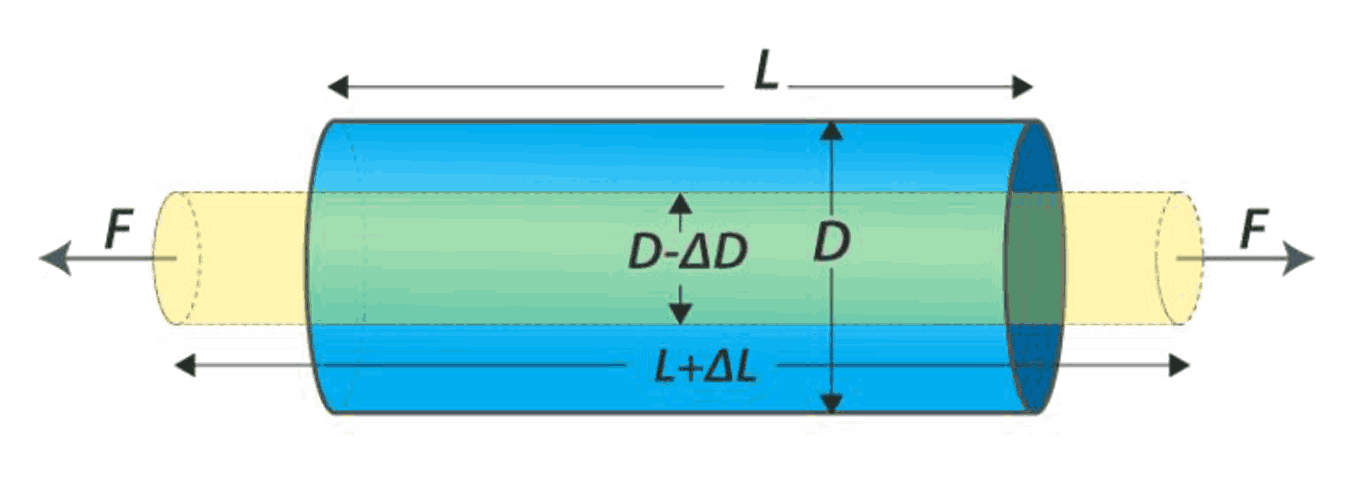Bulk Modulus Bulk modulus is the ratio of normal stress to the volume strain given by the formula,$\text{Bulk modulus}=\dfrac{\text{Normal stress}}{\text{Volumetric strain}}$$\text{Bulk modulus}=\dfrac{F/A}{-\Delta V/V}$$\text{Bulk modulus}=\dfrac{-PV}{\Delta V}$Bulk stress or volume stress can act in solids, liquids and gases and it produces volume strain.Volume strain is the ratio of change in volume to the original volume given by the formula,$\text{Volume strain}=\dfrac{\text{change in volume}}{\text{original volume}}$$\text{Longitudinal strain}=\dfrac{\Delta V}{V}(Image will be uploaded soon) Rigidity modulus Modulus of rigidity is the ratio of tangential stress to the shearing strain given by,\text{Rigidity modulus}=\dfrac{\text{shearing stress}}{\text{shearing strain}}$$\eta=\dfrac{F/A}{\text{x/h}}$Tangential stress or shear stress is developed when the deforming force is applied tangentiallyShearing strain is defined as the angle in radians through which the vertical surface gets turned due to tangential force and its formula is given by,$\text{Shearing strain}=\dfrac{\text{x}}{h}$ The diagram show an example of solid cube undergoing shear stress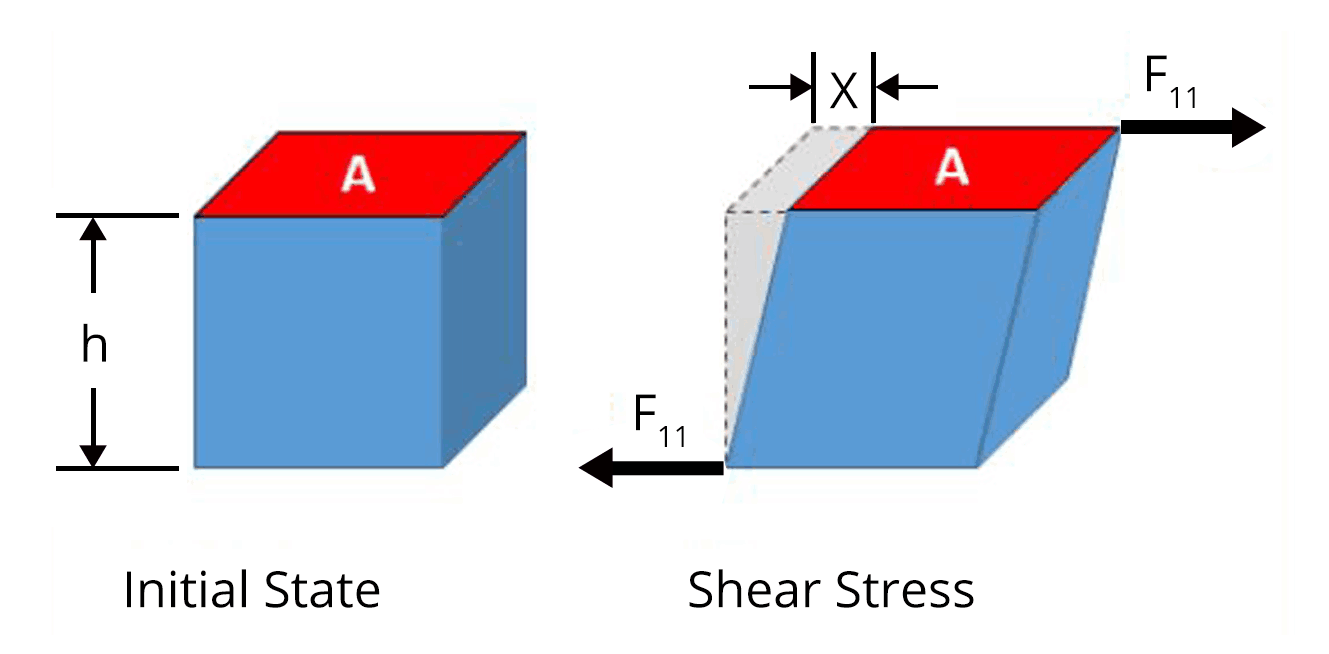Stress-strain curve The behaviour of a material can be studied using stress-strain curve.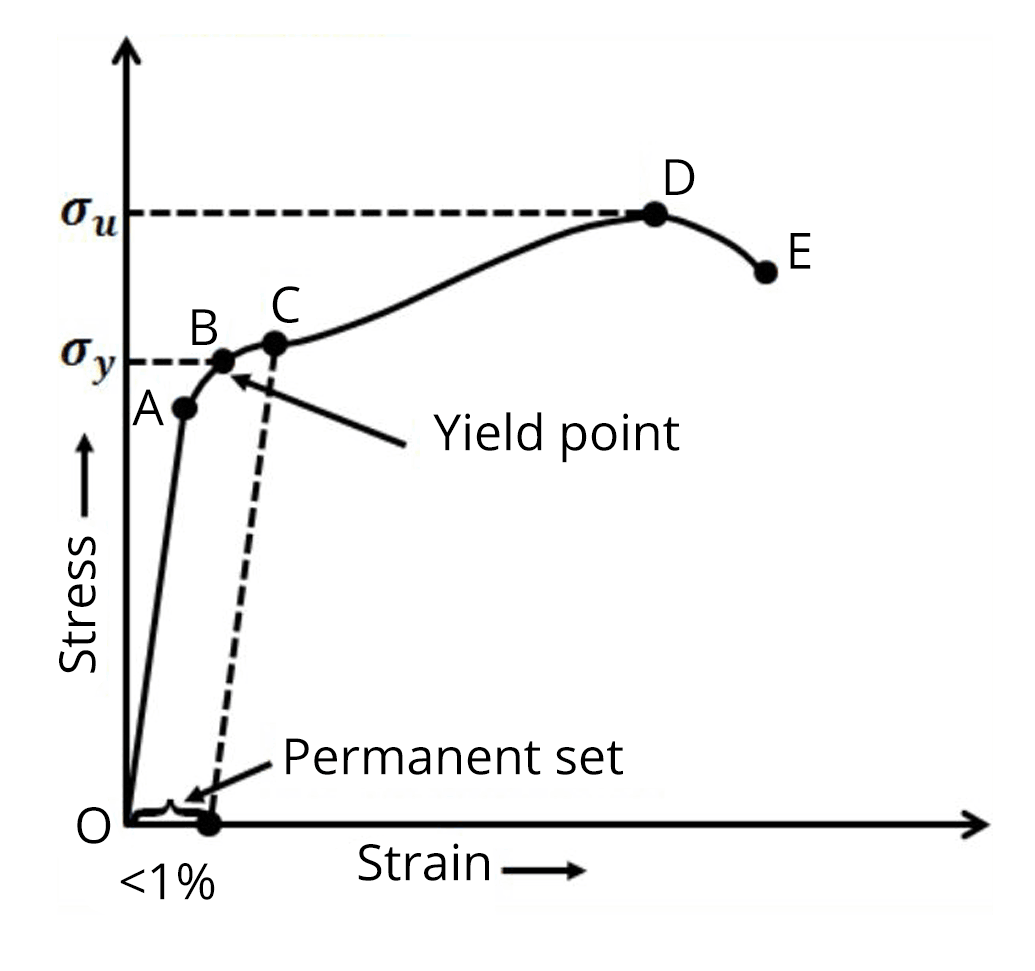The curve obeys Hooke’s law in the region from O to A. According to Hookes law. Stress is proportional to strain.In the stress-strain curve given above, the point A corresponds to proportional limit. The yield point or elastic limit is the point upto which the wire shows elastic behaviour and the point B corresponds to the yield point.The stress corresponding to the yield point is called yield strength.Beyond point B, it loses its elastic behaviour and does not regain its original length even after deforming force is removed.The stress corresponding to point  D is called ultimate strength. Hooke's Law Hooke's Law states that the stress (σ) in a material is directly proportional to the strain (ε) produced in it, within the elastic limit. Mathematically, this can be expressed as:σ = Y * εWhere:σ is the stressε is the strainY is Young's modulus (a material property) Pressure due to a Fluid Column The pressure at any point in a fluid column is determined by the height of the column and the density of the fluid. This concept is crucial in understanding fluid pressure in various applications.The pressure due to a fluid column is given by the following equation:P = ρghwhere:P is the pressureρ is the density of the fluidg is the acceleration due to gravityh is the height of the fluid column Pascal’s Law A change in pressure at any point in a confined incompressible fluid at rest is transmitted undiminished to all points in the fluid.(Image will be uploaded soon)The application of pascal’s law are used in hydraulic lift, hydraulic brakes and press.In hydraulic lift, a small force is converted to a large force having many times the magnitude of the small force. This is a good example of liquid obeying pascal’s law.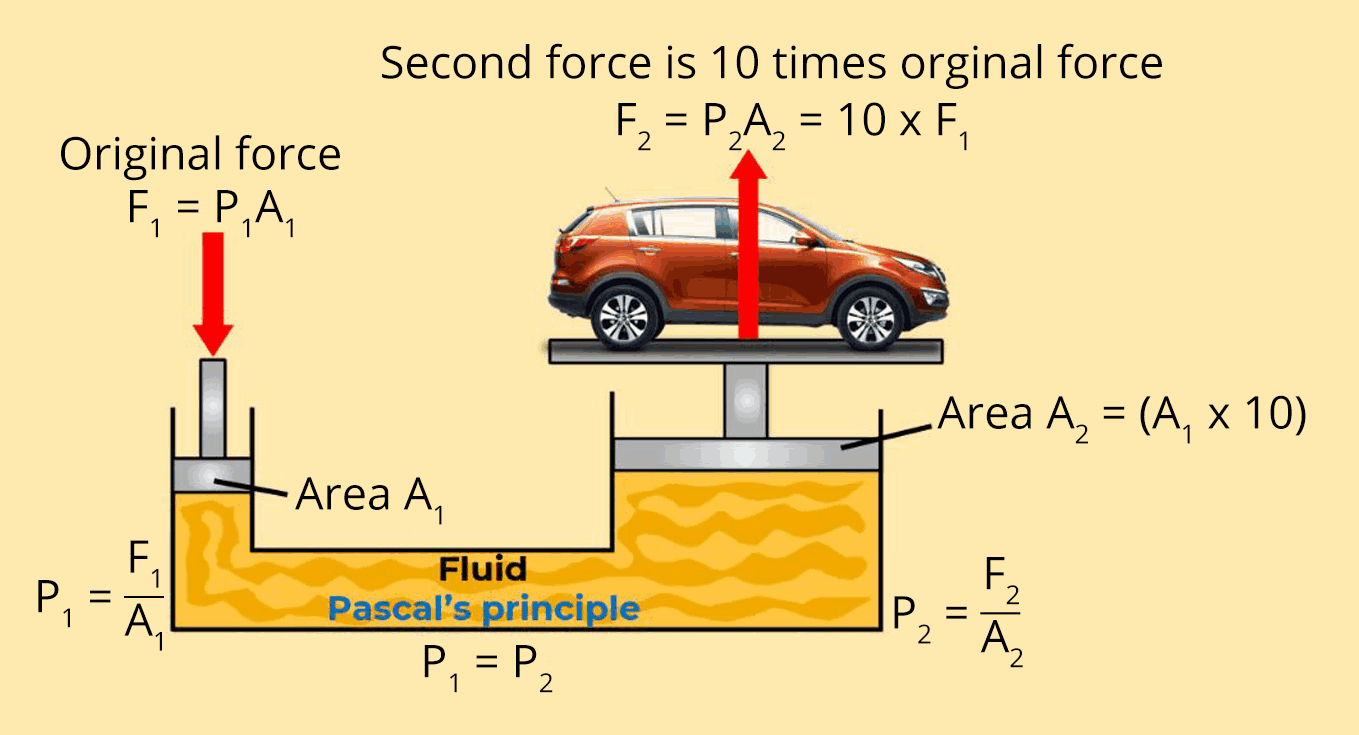Mercurial Barometer Mercurical barometer is used to measure the atmospheric pressure. The fluid pressure corresponds to height of the mercury in the column is equal to the atmospheric pressure.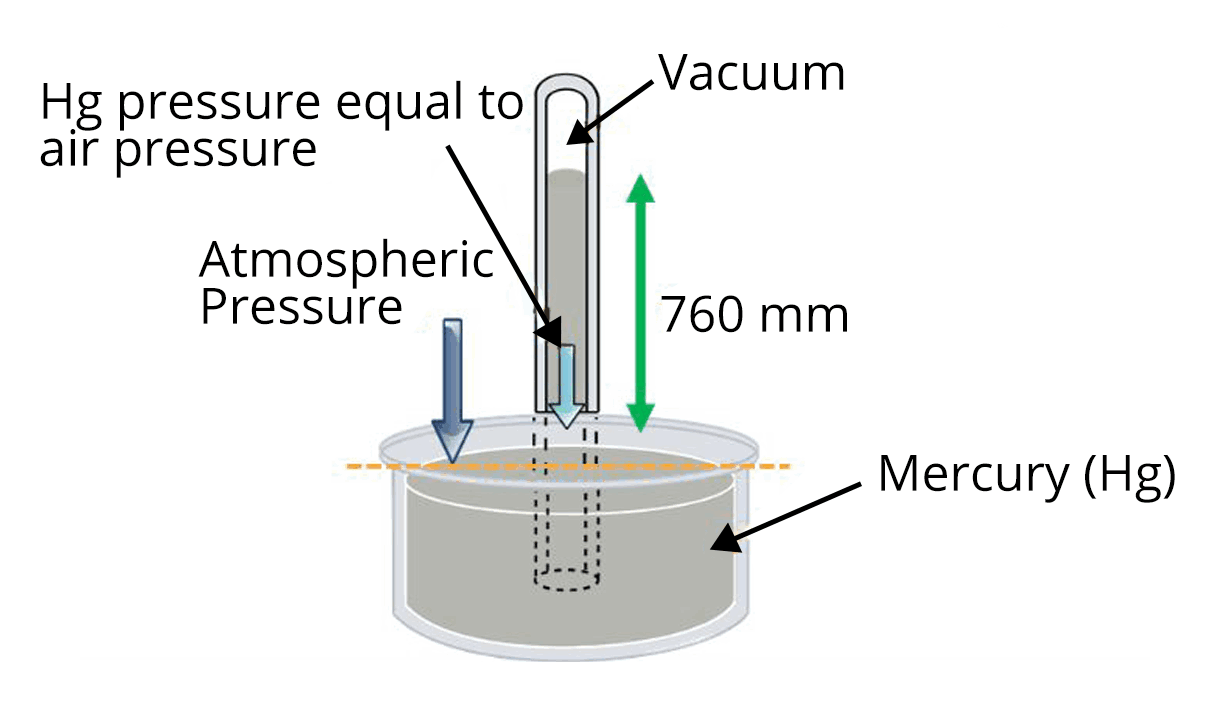Archimedes Principle According to Archimedes principle, whenever a body is fully or partially submerged in liquid, it experiences a net upward force which is equal to the weight of the liquid displaced.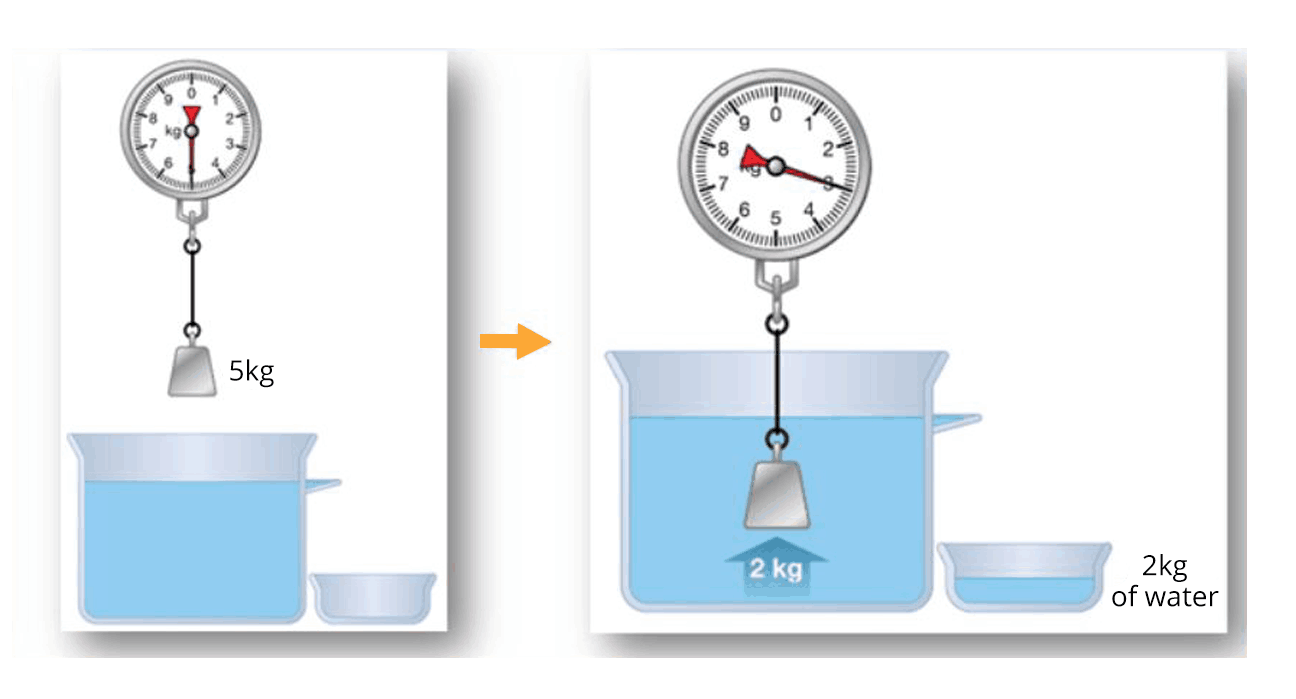The formula to calculate the buoyancy force is given by,$F_B=\rho_l Vg$Where ⍴l is the density of the liquid, V is the volume of the body immersed in the liquid and g is the acceleration due to gravity.A body will float in a liquid if the buoyancy force acting on the body is equal to the weight  of the body. Bernoulli’s Theorem According to Bernoulli’s theorem, the total energy per unit volume of an incompressible fluid always remains constant at any point of the fluid.$P+\rho gh+\dfrac{1}{2}\rho v^2=\text{constant}$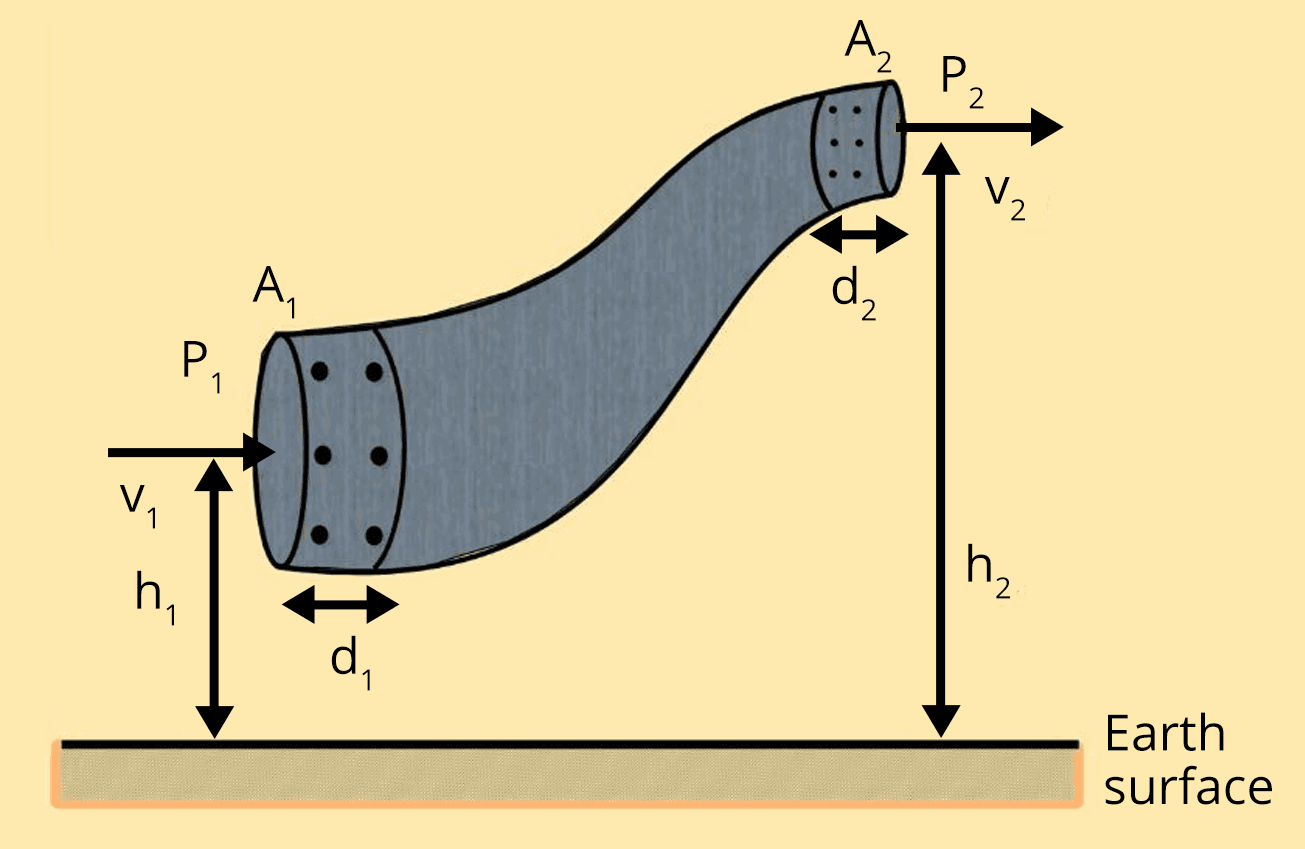$P_1+\rho gh_1+\dfrac{1}{2}\rho v_1^2=P_2+\rho gh_2+\dfrac{1}{2}\rho v_2^2$ Application of Bernoulli’s theorem One of the application of bernouilli’s theorem is the aerodynamic lift.(Image will be uploaded soon)Since the total energy of the fluid remains constant, fluid flowing through upper part of the body will have low pressure compared to the high pressure in the fluid passing through lower side of the body.Due to pressure difference, there is a net force or uplift which helps the body to move up.Another application is when the ball is moving and spinning in air.The ball changes its direction due to pressure difference and this effect is called magnus effect. Viscosity Viscosity is a measure of a fluid's resistance to flow. Understanding viscosity is vital in various applications, such as designing lubricants and understanding the behavior of fluids in pipes. Stoke’s Law When a body falls through a fluid, it drags the layer of the fluid in contact with it, a motion between the different layers of the fluid is set and, as a result the body experiences a retarding force, which is known as Viscous Force.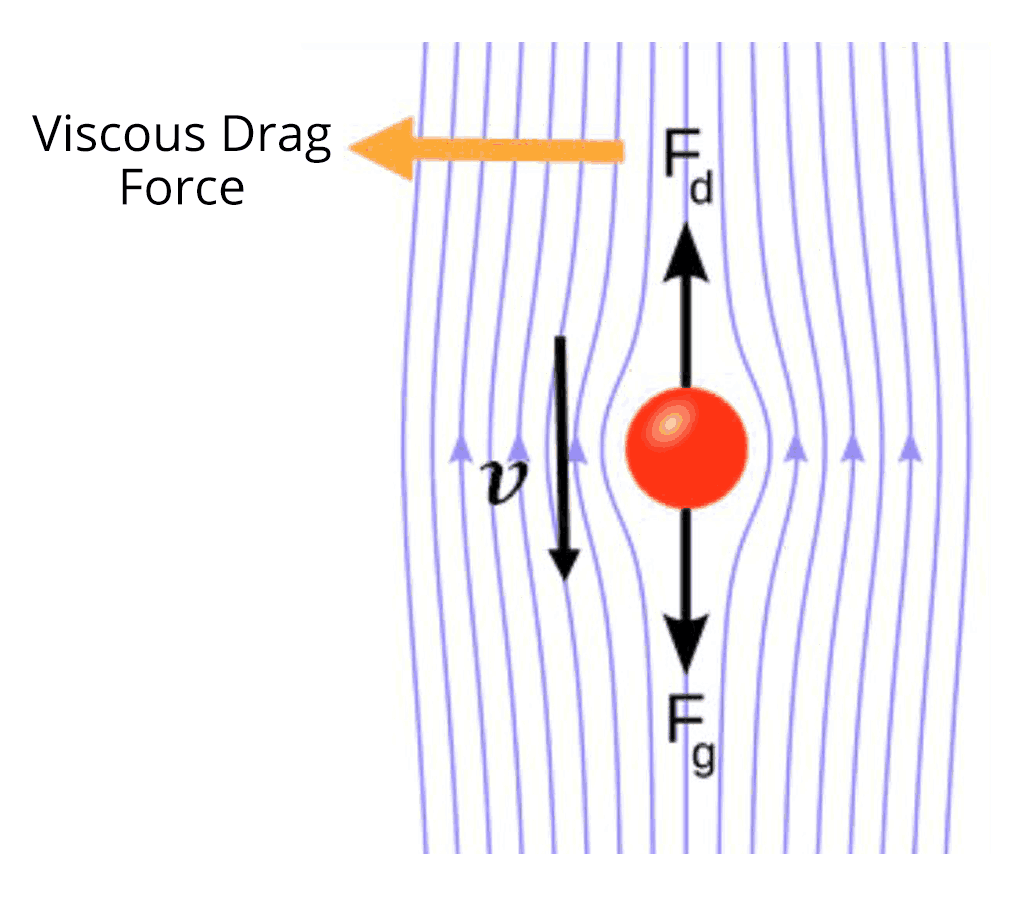$F=6\pi\eta rv$Where η is the coefficient of viscosity, r is the radius of the sphere and v is the velocity of the body.When a body is falling through a liquid, it attains a constant speed when the net force acting on the body becomes zero. This is called terminal velocity. Terminal Velocity, Streamline and Turbulent Flow, Reynolds Number Terminal Velocity: Terminal velocity is the constant velocity attained by an object when the drag force equals the gravitational force. It is a critical concept in understanding fluid dynamics.Streamline and Turbulent Flow: Understanding the difference between streamline and turbulent flow is important in fluid mechanics, particularly in designing efficient transport systems.Reynolds Number: The Reynolds number is a dimensionless quantity that characterizes the flow regime of a fluid. It helps in predicting whether a flow will be laminar or turbulent. Surface tension and surface energy Surface tension is net effect of cohesive forces in downward direction on any surface.(Image will be uploaded soon)The unit of surface tension is N/m.Surface energy is the potential energy contained by  the molecules of the liquid surface due to which they stay on the surface against inward force of attraction by other molecules.The magnitude of surface energy per unit area and surface tension are equal.The relationship between surface tension ans surface energy is given by,$\text{Surface tension}=\dfrac{\text{Surface energy}}{\text{Area}}$ Angle of Contact The angle of contact between a liquid and a solid surface is crucial in understanding the shape of liquid surfaces in various situations. Heat Transfer - Conduction, Convection, and Radiation Heat transfer occurs through conduction (direct contact), convection (through fluid movement), and radiation (through electromagnetic waves). These principles are essential in various fields, from cooking to the design of spacecraft heat shields.Explore further elaboration on the distinctions between Conduction, Convection, and Radiation to gain a clearer understanding. Newton's Law of Cooling Newton's Law of Cooling describes the rate of change of temperature of an object in contact with a medium of a different temperature. This law has practical applications in fields such as meteorology and engineering.Exploring the diverse aspects of solids and liquids, one can delve into topics such as their mechanical behavior, fluid dynamics, thermal properties, and more.

## Application of Surface Tension

• Raindrops: Raindrops are spherical because of surface tension. This helps the raindrops to fall faster through the air.

• Bubbles: Bubbles are spherical because of surface tension. This also helps the bubbles to float in the air.

• Plants: Plants absorb water from the soil through their roots. The water is transported up the stem and leaves through capillary rise.

• Wicks: The wicks in candles and pens work by capillary rise. The wick draws the wax or ink up from the reservoir and to the tip of the wick.

• Insects: Some insects, such as water striders, can walk on the surface of water because of surface tension.

• Cleaning: Surface tension is used in some cleaning products to help them to remove dirt and grease.

## Heat, Temperature, and Thermal Expansion

### Heat and Temperature:

Heat is the transfer of thermal energy, while temperature is a measure of the average kinetic energy of particles in a substance. Understanding the relationship between heat and temperature is fundamental to thermodynamics.

### Thermal Expansion:

Thermal expansion is the property of matter by which it expands or contracts when exposed to changes in temperature. This phenomenon has practical implications in various industries, such as construction and engineering.

### Specific Heat Capacity and Calorimetry

Specific heat capacity is the amount of heat required to raise the temperature of a unit mass of a substance by one degree. Calorimetry is the science of measuring heat changes in chemical processes and has applications in various fields, including chemistry and food science.

### Change of State and Latent Heat:

Change of State:

A change of state is a physical change in which a substance changes from one phase to another. The three most common phases of matter are solid, liquid, and gas. Other phases, such as plasma and Bose-Einstein condensates, also exist.

Latent Heat:

Latent heat is the energy that is absorbed or released during a change of state. It is the energy that is required to break the intermolecular bonds in a solid or liquid to form a gas, or to form the intermolecular bonds in a gas or liquid to form a solid.

Types of Latent Heat:

• Latent heat of fusion is the energy that is absorbed or released when a substance changes from solid to liquid or vice versa. For example, the latent heat of fusion of ice is 334 J/g. This means that it takes 334 J of energy to melt 1 gram of ice at its melting point.

• Latent heat of vaporization is the energy that is absorbed or released when a substance changes from liquid to gas or vice versa. For example, the latent heat of vaporization of water is 2260 J/g. This means that it takes 2260 J of energy to vaporize 1 gram of water at its boiling point.

Applications of Latent Heat:

• Refrigeration and air conditioning: Latent heat of vaporization is used to absorb heat from the environment in refrigerators and air conditioners.

• Cooking: Latent heat of vaporization is used to cook food in steamers and pressure cookers.

• Power generation: Latent heat of vaporization is used to generate steam in power plants.

### List of Important Formulas for Properties of Solids and Liquids Chapter

 Sl. No. Name of the Concept Formula 1. Work done in stretching a strain Work done in stretching a wire having young’s modulus(Y) under a force (F) is given by the formula,$W=\dfrac{1}{2}\dfrac{YA\Delta l^2}{l}$ 2. Thermal stress on a rod fixed between two rigid supports. $\text{Thermal stress }=Y\alpha\Delta T$Where ⍺ is the coefficient of linear expansion and ΔT is the change in temperature 3. Force constant of the wire having a length(l) and area of cross section(A) $K=\dfrac{YA}{l}$ 4. Depression produced in the beam of bridge (Image will be uploaded soon)$\delta=\dfrac{Wl^3}{4Ybd^3}$ 5. Energy stored per unit volume of wire $U_V=\dfrac{1}{2}\times\text{stress}\times\text{strain}$$U_V=\dfrac{1}{2}\times Y\times\text{strain}^2$ 6. Variation Of Pressure With Depth Inside The Fluid $P_2-P_1=\rho gh$(Image will be uploaded soon) 7. Equation of continuity $A_1V_1=A_2V_2$Where A1 and A2 are the area of cross section of the pipe at the two ends and V1 and V2 are the corresponding velocity of the fluid.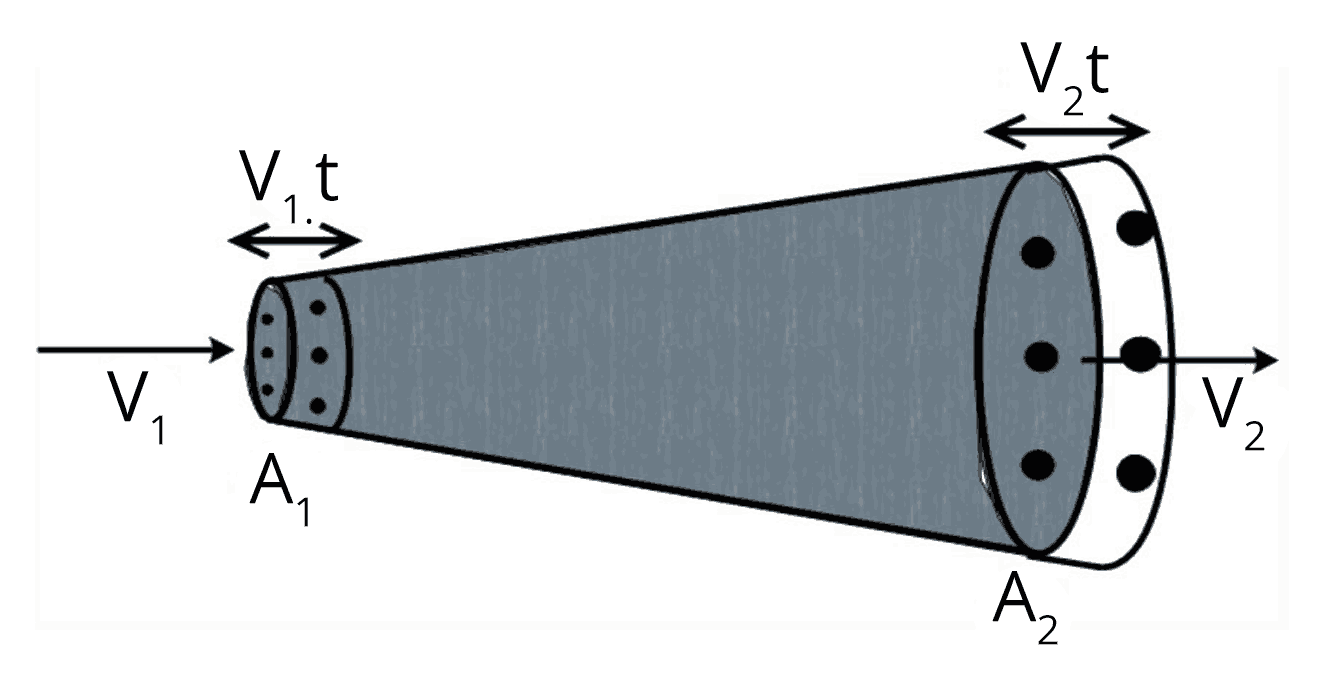8. Velocity of efflux Velcoity of water flowing out from the container is given by,$v=\sqrt{2gh}$(Image will be uploaded soon) 9. Excess pressure inside a liquid drop $p=\dfrac{2S}{r}$Where S is the surface tension and r is the radius of the drop 10. Excess pressure inside a liquid drop $p=\dfrac{4S}{r}$Where S is the surface tension and r is the radius of the bubble. 11. The height of capillary rise inside a tube $h=\dfrac{2S\cos\theta}{\rho rg}$(Image will be uploaded soon)

### JEE Main Properties of Solids and Liquids Solved Examples

1. When a load of 5 kg is hung on a wire, then the extension of 3 m takes place. The work done will be,

Ans:

The mass of the load hung on the wire, m = 5 kg

The force acting on the wire is calculated as ,

$F=mg$

$F=5\times9.8$

$F=49~N$

We can calculate the work done in extending the wire by using the formula given below,

$W=\dfrac{1}{2}F\Delta l$

$W=\dfrac{1}{2}\times 49\times 3$

$W=73.5~J$

Key Point: The work done in extending the wire is half the product of the force acting on the wire and the extension of the wire.

1. In a capillary tube, water rises by 1.2 mm. The height of water will rise in another capillary tube having half the radius of the first will be ____.

Ans:

The formula to calculate the capillary rise of first tube is given by,

$h_1=\dfrac{2S\cos\theta}{\rho rg}$

$1.2~mm=\dfrac{2S\cos\theta}{\rho rg}$...(1)

The capillary rise of the water in the second tube is given by,

$h_2=\dfrac{2S\cos\theta}{\rho (r/2)g}$

$h_2=\dfrac{4S\cos\theta}{\rho rg}$.....(2)

Divide the equation (2) by equation (1) to calculate the height of capillary rise in second tube.

$\dfrac{h_2}{1.2~mm}=\dfrac{\dfrac{4S\cos\theta}{\rho rg}}{\dfrac{2S\cos\theta}{\rho rg}}$

$h_2=2\times2~mm$

$h_2=4~mm$

Key Point:  The surface tension and the angle of contact in both tubes are equal since the same liquid is used in both tubes.

### Previous Year Questions from JEE Exam

1. A hydraulic press can lift 100 kg when a mass ‘m’ is placed on the smaller piston. It can lift _______ kg when the diameter of the larger piston is increased by 4 times and that of the smaller piston is decreased by 4 times keeping the same mass ‘m’ on the smaller piston. (JEE 2021)

Ans:

Applying Pascal’s law, the pressure acting on smaller piston due to mass m is equal to the pressure acting on the larger piston.

Let A1 and A2 be the area of the smaller piston and larger piston respectively.

$\dfrac{mg}{A_1}=\dfrac{100g}{A_2}$

$\dfrac{A_1}{A_2}=\dfrac{m}{100}$...(1)

Now, let M be the mass that hydraulic press can lift after changing the area of cross section of both pistons.

Again applying pascal’s law,

$\dfrac{mg}{(A_1/16)}=\dfrac{Mg}{(16A_2)}$

$\dfrac{A_1}{A_2}=256\dfrac{m}{M}$...(2)

Using  equation (1) and equation (2), we can calculate the value of M.

$\dfrac{m}{100}=256\dfrac{m}{M}$

$M=25600~kg$

Therefore, the new mass it can lift is 25600 kg

Trick:  The pressure acting on both the piston are equal according to the Pascal’s law.

1. A uniform metallic wire is elongated by 0.04 m when subjected to a linear force F. The elongation, if its length and diameter is doubled and subjected to the same force will be ________ cm. (JEE 2021)

Ans:

The formula to calculate the young’s modulus of the wire is given by,

$Y=\dfrac{Fl}{A\Delta l_1}$....(1)

When the diameter is doubled, the area of cross section of the wire becomes four times initial area of cross section.

When the same force is applied after the length and diameter is doubled, then formula for young’s modulus is given by,

$Y=\dfrac{F(2l)}{(4A)\Delta l_2}$

$Y=\dfrac{Fl}{2A\Delta l_2}$....(2)

Using equation (1) and (2),

$\dfrac{Fl}{A\Delta l_1}=\dfrac{Fl}{2A\Delta l_2}$

$\Delta l_2=\dfrac{\Delta l_1}{2}$

$\Delta l_2=\dfrac{0.04}{2}$

$\Delta l_2=0.02~m=2~cm$

Therefore, the new extension will be 2 cm.

Trick: Young’s modulus deos not depend on the dimensions on the wire and only depends onthe material.

### Practice Questions

1. An iceberg of density 900 kg/m3 is floating in water of density 1000 kg/m3. The percentage of the volume of ice cube outside the water is ? (Ans: 10 %)

2. A wind with speed 40 m/s blows parallel to the roof of a house. The area of the roof is 250 m2. Assuming that the pressure inside the house is atmospheric pressure, the force exerted by the wind on the roof will be (Ans: 2.4✕105 N)

## JEE Main Physics Properties of Solids and Liquids Study Materials

Here, you'll find a comprehensive collection of study resources for Properties of Solids and Liquids designed to help you excel in your JEE Main preparation. These materials cover various topics, providing you with a range of valuable content to support your studies. Simply click on the links below to access the study materials of Properties of Solids and Liquids and enhance your preparation for this challenging exam.

 JEE Main Properties of Solids and Liquids Study Materials JEE Main Properties of Solids and Liquids Notes JEE Main Properties of Solids and Liquids Important Questions JEE Main Properties of Solids and Liquids Practice Paper

## JEE Main Physics Study and Practice Materials

Explore an array of resources in the JEE Main Physics Study and Practice Materials section. Our practice materials offer a wide variety of questions, comprehensive solutions, and a realistic test experience to elevate your preparation for the JEE Main exam. These tools are indispensable for self-assessment, boosting confidence, and refining problem-solving abilities, guaranteeing your readiness for the test. Explore the links below to enrich your Physics preparation.

 JEE Main Physics Study and Practice Materials JEE  Main Physics Previous Year Question Papers JEE Main Physics Mock Test JEE Main Physics Formula JEE Main Sample Paper JEE Main Physics Difference Between

### Conclusion

In this article, we dive into the world of Solids and Liquids, unraveling their fascinating properties. We'll explore the characteristics that make solids and liquids unique, like their ability to flow or maintain a fixed shape. You can grasp the concepts easily with our clear explanations and find solutions to common questions. All the information you need is right here, from understanding the basics to tackling exam questions. Plus, our PDF resources are free for you to download. This will undoubtedly boost your confidence for any upcoming exams!

## FAQs on Properties of Solids and Liquids Chapter - Physics JEE Main

1. How many questions are asked from properties os solids and liquids in JEE?

About 2-3 questions from this chapter are asked in JEE exam every year which corresponds to around 8-12 marks in the JEE Main exam.

2. Is the chapter properties of solids and liquids a tough chapter?

As far as teh JEE main exam is concerned, properties of solids and liquids is a moderate difficult chapter. But it is a vast chapte which consists of more theories and concepts and related formulas. But with proper learning and practices, all the concepts in this chapter can be learned without any difficulty.

3. How to get a good score in JEE Main to get into the best NITs?

To get into best NITs in the nation, we have to crack the JEE Main exam with good scores. Even though JEE Main is a very competitive exam, we can score good marks if we understand the concepts in depth and pratice lots of questions and finally doing all the previous year question papers without any fail.

### Repeaters Course for JEE 2022 - 23## Notice board

JEE News
JEE Blogs